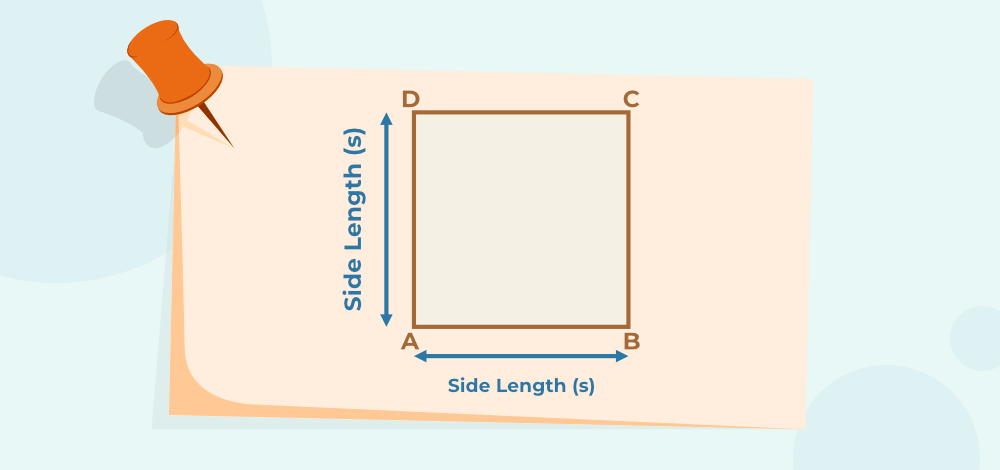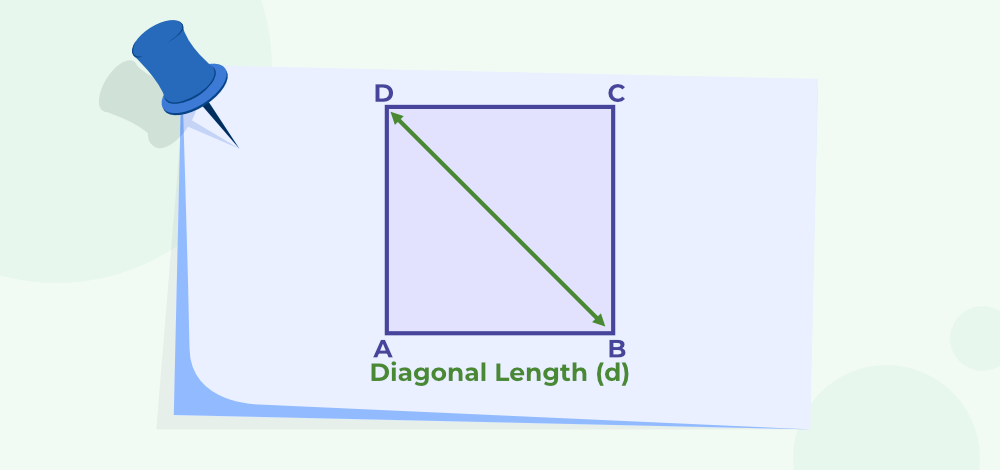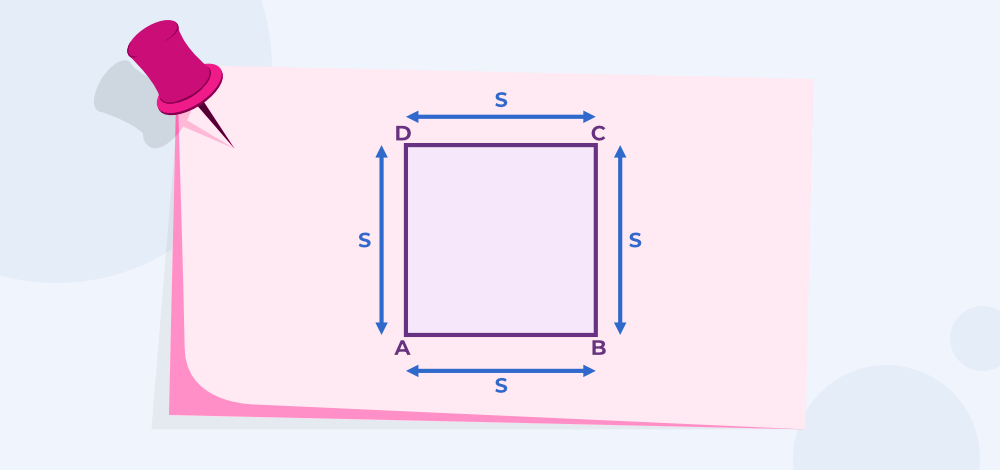# Area of a Square

Area of a Square or area of any figure is defined as the space occupied by it in 2-D space. So, Area of the square is defined as the space enclosed by the boundary of the square. The measurement of the area is done in square units. The SI unit for measurement of the area is m2.

For finding areas of various figures, several pre-defined formulas are used, in this article, we will study the formulas for finding the area of the square.

## What is Area of Square?

Space enclosed inside the boundaries of any figure is called the area of the figure. It is a physical quantity that gives us the idea of how much space is covered by an object.

Square is a Two-Dimensional (2D) figure which has 4 sides of all equal lengths. The area of a square concept comes under the topic of mensuration which deals with the measurements of Two Dimensional and Three Dimensional figures. Length, Perimeter, Area, Volume, etc. come under the measurements of a figure.

The area of the square is calculated by multiplying its sides by its sides, i.e. finding its sides square.

### Area of Square Definition

The area is the region inside the boundaries of an object. The area of a square is defined as the number of square units needed to fill the square. To calculate the square area we need to know the length of any of its sides. The area of the square can be calculated by squaring the length of any of its sides.

## Area of Square Formula

The various formulas for finding the area of a square are listed below,

Area of Square = Side2 unit2Area can be measured in various units, some of the conversions for changing standard units of the area to other desired units are given below:

1 m2 = 10000 cm2

1 km2 = 1000000 m2

If required in calculation we can find the perimeter of the square by the given formula

Perimeter of square = 4 × sides units

### Example: What is the area of a square if each side of length is 4cm?

Solution:

Given

Side length (s) = 4cm

Area of square = s2

= 42

= 16cm2

Area of square with side length 4cm is 16cm2.

## Area of Square using DiagonalsArea of Square when the diagonal length is given,

Area = (1/2) × d2

where,

d is the length of diagonal.

### Example: Find the area of a square if the length of the diagonal is 6cm.

Solution:

Given

Diagonal length (d) = 6cm

Area = (1/2) × d2

= (1/2) × 62

= 36/2

= 18cm2

Area of square is 18cm2.

## Area of Square Using PerimeterWe can find an area even if the perimeter of a square is given.

The formula of the perimeter of a square = 4 × side

From the above formula, we can find the side length by dividing the Perimeter by 4.

Side length(s) = Perimeter/4

Using side length we can find the area of the square by using the formula Area = side ×  side.

### Example: Find the Area of the Square if theperimeter of a square is 36 cm.

Solution:

Given

Perimeter=36 cm

So, Side length=perimeter/4

Side(s) = 36/4

= 9 cm

From the side length we can calculate area of square by

Area = Side2
= 92
= 81 cm2

Area of square with perimeter 36 cm  is 81 cm2.

## How to Find Area of Square?

Area of square can be calculated if the dimensions of the square are known. We can calculate the area of the square by various formulas depending on the initial values that are given.

Follow the steps given below to find the area of the square.

Step 1: Note the dimension of the square given. For example to find the area of a square with a side of 10 m. The given dimension (side) is 10 m.

Step 2: Use the area of the Square formula. As Area of Square = (Side)2. For given example,

Area of Square = (10)2

Step 3: Simplify the value obtained in step 2. For given example,

Area of Square = (10)2 = 100

Step 4: Add unit2 as the unit to the answer obtained in step 3 to get the final answer. For given example,

Area of Square = 100 m2

Related Articles:

## Solved Examples on Area of Square

### Example 1: Find the Area of the Square if theperimeter of a square is 64cm.

Solution:

Given

Perimeter=64cm

So, Side length=perimeter/4

Side(s) = 64/4

= 16cm

From the side length we can calculate area of square by

Area =Side2

= 162

= 256cm2

Area of square with perimeter 64cm  is 256cm2.

### Example 2: Find the area of a square if the length of the diagonal is 12cm.

Solution:

Given

Diagonal length (d) = 12 cm

Area = (1/2) × d2

= (1/2) × 122

= 144/2

= 72 cm2

Area of square is 72 cm2.

### Example 3: The length of each side of a square is 5cm and the cost of painting it is Rs. 5 per sq. cm. Find the total cost to paint the square.

Solution:

Given

Side length (s) = 5cm

Area of square = s2

= 52

= 25 cm2

For 1 sq.cm cost of painting is Rs 5.

Total Cost of painting the 25sq cm= 25 × 5 = Rs125

### Example 4: A floor that is 60 m long and 30 m wide is to be covered by square tiles of side 6 m. Find the number of tiles required to cover the floor.

Solution:

Length of the floor = 60 m

Breadth of the floor = 30 m

Area of floor = length × breadth

= 60 m × 30 m = 1800 sq. m

Length of one tile = 6 m

Area of one tile = side ×side

= 6 m  × 6 m = 36 sq. m

No. of tiles required = (area of floor)/(area of one tile)

= 1800/36

= 50 tiles.

Total tiles required is 50.

### Example 5: What is the Area of aSquare if theperimeter of a square is 24 cm?

Solution:

Given,

Perimeter = 24cm

So, Side length = perimeter/4

Side(s) = 24/4

= 6cm

From the side length we can calculate area of square by

Area = Side2

= 62

= 36cm2

Area of square with perimeter 24 is 36cm2.

## FAQs on Area of Square

### Q1: What is the Area of the square?

Area of a square is defined as the total number of units of a square that is enclosed by the boundary of a square. i.e. it is defined as the space occupied by the square in the 2-D plane.

### Q2: What is the Formula for the area of a square?

A square is a quadrilateral with all four sides equal. Its area can be calculated with formula Sides square, i.e.

Area of Square = side × side square units.

### Q3: What is the unit for measuring the area of a square?

Area of square is measured in square units i.e. square m, square cm, etc.

### Q4: What is the area of the square when a diagonal is given?

Suppose the diagonal of a square is given, then the formula to find the area of a square is given by:

Area = (½) × d2 square units

where,
d” is the diagonal

### Q5: How to find the Area of the Square Pyramid?

The area of square is found using the formula

Area = (s)2

where
s is the side of the square.

It is measures in m2, cm2, and other units.

### Q6: How the area of the square is derived?

The area of the square is derived as,

we know that area of the square when the diagonal is given,

Area = (½) × d2 square units

We know that all the sides in the square are equal say (a), then by using Pythagoras theorem,

d = √2(a)

then put this in the above formula we get,

Area = (½) × (√2a)2

Area = ½ × 2a2 = a2

Thus, the area of the square is derived.

### Q7: How to find the area of a square pyramid?

The area of a square pyramid is calculated using the formula,

Area of square = a2 + 2a√[(a/2)2 + h2]

where,
a is the base length of the pyramid
h is the height of the pyramid

Whether you're preparing for your first job interview or aiming to upskill in this ever-evolving tech landscape, GeeksforGeeks Courses are your key to success. We provide top-quality content at affordable prices, all geared towards accelerating your growth in a time-bound manner. Join the millions we've already empowered, and we're here to do the same for you. Don't miss out - check it out now!

Previous
Next